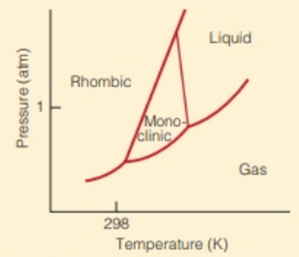Chapter 6, Problem 6.67E

Chapter
Section
Textbook Problem

The phase diagram for elemental sulfur is shown in Figure 6.18.Figure 6.18 Phase diagram for elemental sulfur.(a) How many allotropes are shown? (b) What is the stable allotrope of sulfur under normal conditions of temperature and pressure? (c) Describe the changes to sulfur as its temperature is increased from 25 ° C while at 1   atm pressure.

(a)

Interpretation Introduction

Interpretation:

The number of allotropes of sulfur shown in the given phase diagram is to be stated.

Concept introduction:

Phase diagram represents the different physical states of a substance at different values of temperature and pressure. The lines or the curves in a phase diagram represent the phase transitions.

Explanation

The phase diagram of elemental sulfur,

(b)

Interpretation Introduction

Interpretation:

The stable allotrope of sulfur at normal conditions of temperature and pressure is to be stated.

Concept introduction:

Phase diagram represents the different physical states of a substance at different values of temperature and pressure. The lines or the curves in a phase diagram represent the phase transitions.

(c)

Interpretation Introduction

Interpretation:

The changes in sulfur as its temperature is increased from 25°C at 1atm pressure are to be stated.

Concept introduction:

Phase diagram represents the different physical states of a substance at different values of temperature and pressure. The lines or the curves in a phase diagram represent the phase transitions.

Still sussing out bartleby?

Check out a sample textbook solution.

See a sample solution

The Solution to Your Study Problems

Bartleby provides explanations to thousands of textbook problems written by our experts, many with advanced degrees!

Get Started

Find more solutions based on key concepts